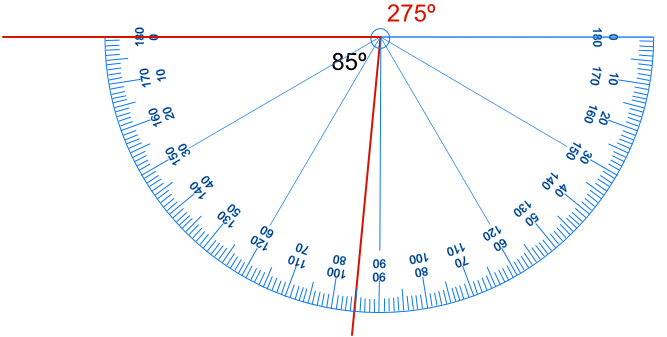Drawing Angles

## Drawing Angles

Drawing angles is similar to measuring angles.

Ensure that the centre of the protractor is positioned to be on the point of the angle. Make sure that the baseline of the protractor lies along the first line of the angle.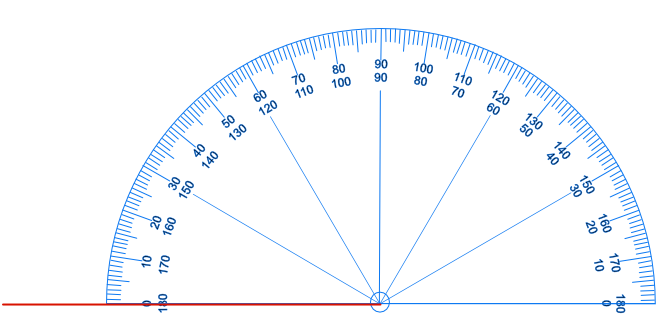Check which scale is being used on the protractor: in this case, the zero lying on the line is the zero from the outer scale.

Read around the scale to the desired angle: for example, 48º: Make a mark on the paper at that point on the scale.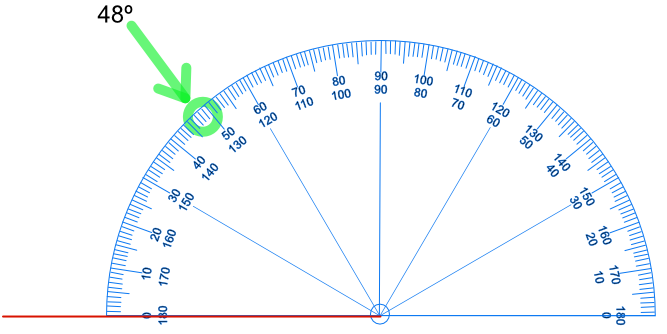Draw a line through the mark to the point of the angle. Write the value of the angle on the angle.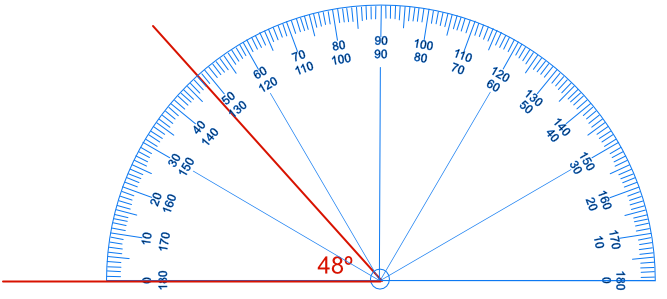## Example 1

Draw an angle of 122º

Set the protractor with the centre of the protractor on the point. Read from the zero scale that lies on the line (the outer scale). Read round to mark 122º, and draw a line from the point of the angle through this point.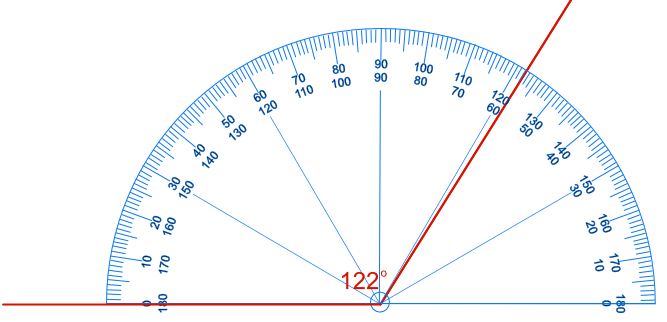## Example 2

Draw an angle of 275º.

Most protractors measure up to 180º. The required angle is greater than that.

Draw the angle that would complete the circle: 360º - 275º = 85º. Measure an angle of 85º. The angle of 275º is that part that was not measured.